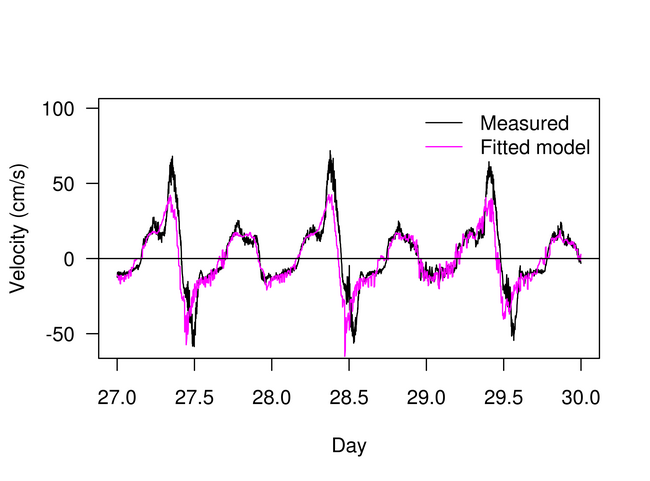Figure 4:View Original Image at Full Size

A comparison of the measured and predicted water velocities at the 'velocity measurement' site (Figure 1b), using Equation 1. The water level data used for prediction was measured near the seaward edge of the channel (Figure 1b). The first day of velocity and stage data was used to estimate F(h), using linear regression. F(h) was estimated as a third order polynomial, because increasing the order of the polynomial did not significantly change the predictions.

Image 16444 is a 4800 by 6480 pixel PNG